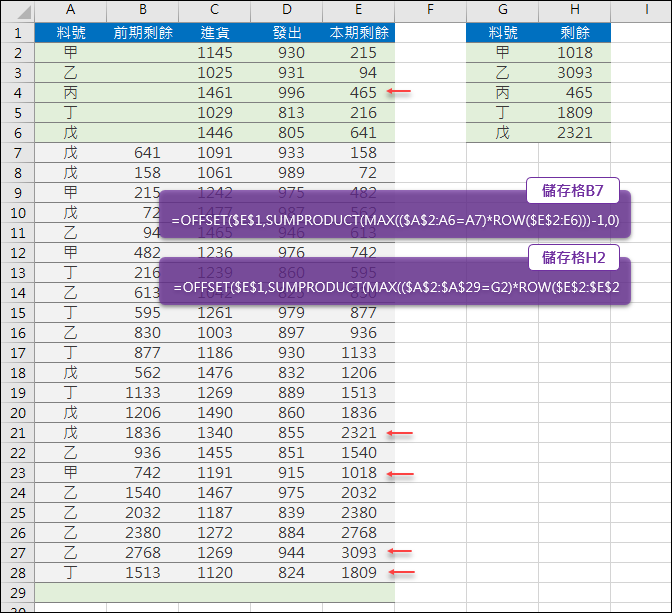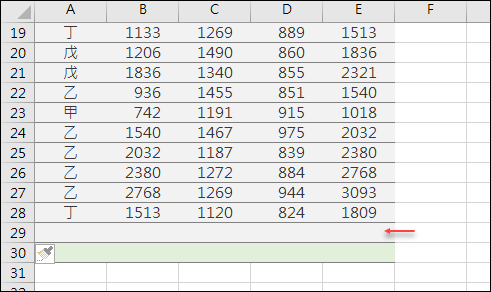# Excel-在持续新增的资料中找出各项最后一个数值(SUMPRODUCT,OFFSET)【公式设计与解析】

1. 计算前期剩余

=OFFSET(\$E\$1,SUMPRODUCT(MAX((\$A\$2:A6=A7)*ROW(\$E\$2:E6)))-1,0)

(1) \$A\$2:A6=A7

SUMPRODUCT 函数中找出符合条件的阵列（由单元格A2起始至单元格A6的范围中和单元格A7相同者），传回 TRUE／FALSE 阵列。

(2) (\$A\$2:A6=A7)*ROW(\$E\$2:E6)

(3) MAX((\$A\$2:A6=A7)*ROW(\$E\$2:E6))

SUMPRODCUT 函数中，利用 MAX 函数找出第(2)式传回的运算结果之最大值，即为最大的列号，也就是符合料号的最后一个单元格。

(4) OFFSET(\$E\$1,SUMPRODUCT(第(3)式)-1,0)

2. 计算本期剩余

=OFFSET(\$E\$1,SUMPRODUCT(MAX((\$A\$2:\$A\$29=G2)*ROW(\$E\$2:\$E\$29)))
-1,0)

3. 持续新增资料但不改公式• 1 Responses to “Excel-在持续新增的资料中找出各项最后一个数值(SUMPRODUCT,OFFSET)”